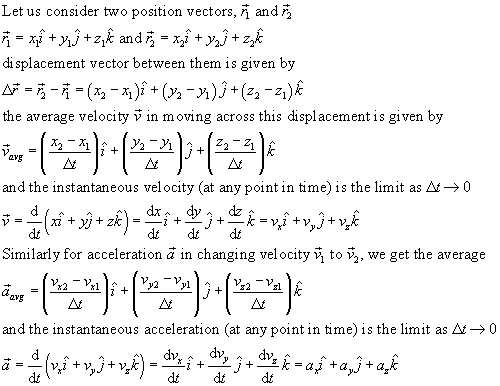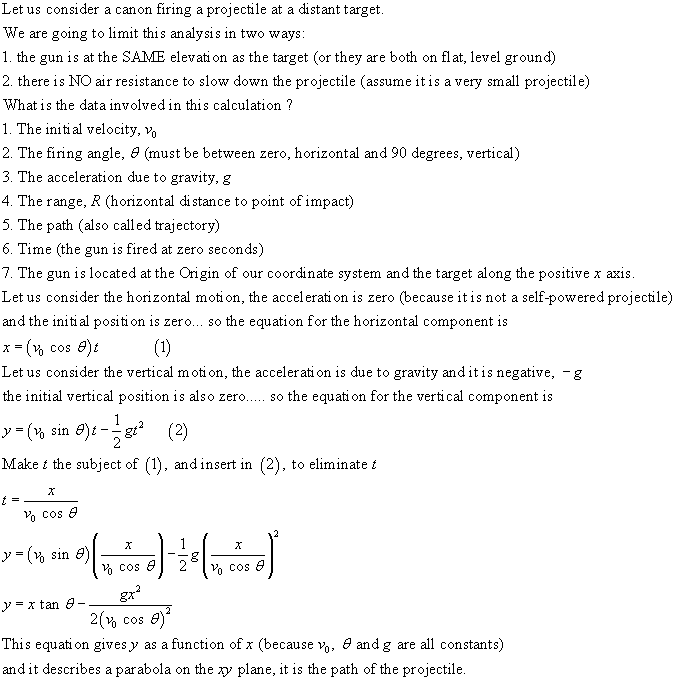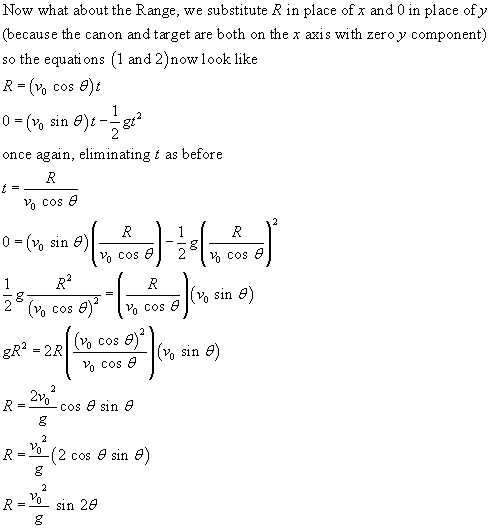## Motion in two and three dimensions

To properly understand 2-D and 3-D motion we have to apply the equations of motion to each dimension INDIVIDUALLY, and since the equations are linked by time, we must then solve these equations simultaneously. Solutions to problems are done by writing a set of equations (one for each dimension) and then solving them simultaneously according to the normal rules of mathematics.P10C Homepage